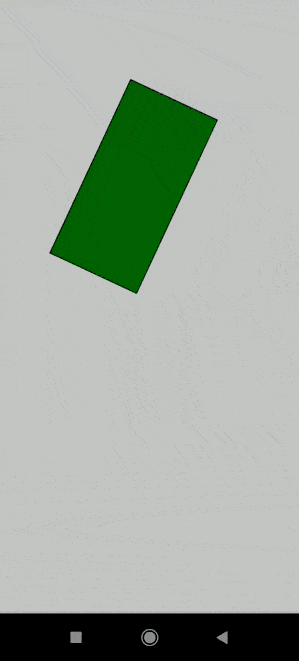GFG App
Open AppBrowser
Continue

# p5.js deviceOrientation variable

The deviceOrientation variable contains the orientation of the device. The value of this variable in the code will either be set landscape or portrait. It is used in mobile devices for detecting the orientation and can be used to change the sketch for another orientation if required. When no data is available, the variable will be set to undefined.

Syntax:

`deviceOrientation`

Example:

## Javascript

 `// Define variables that would hold the ` `// x, y and z values of orientation ` `let x = 0; ` `let y = 0; ` `let z = 0; ` ` `  `function` `setup() { ` ` `  `  ``createCanvas(400, 400); ` `  ``if` `(window.DeviceOrientationEvent) { ` ` `  `    ``// Add event listener to the function when ` `    ``// the device orientation changes ` `    ``window.addEventListener(``"deviceorientation"``,  ` `      ``onOrientationChange); ` `  ``} ` `} ` ` `  `function` `draw() { ` `  ``background(255, 255, 255); ` `  ``angleMode(DEGREES); ` ` `  `  ``rectMode(CENTER); ` `  ``translate(width / 2, height / 2); ` ` `  `  ``// Rotate on the basis of the y-axis ` `  ``rotate(y); ` ` `  `  ``let c = color(``"green"``); ` `  ``fill(c); ` ` `  `  ``// Draw a rectangle and fill the above ` `  ``rect(0, 0, 100, 200); ` `} ` ` `  `// Assign the x, y, z variables to ` `// the event details ` `function` `onOrientationChange(e) { ` `  ``x = e.x; ` `  ``y = e.y; ` `  ``z = e.z; ` ` `  `  ``console.log(``"X:"``, x, ``"Y:"``, y, ``"Z:"``, z); ` `}`

Output:My Personal Notes arrow_drop_up